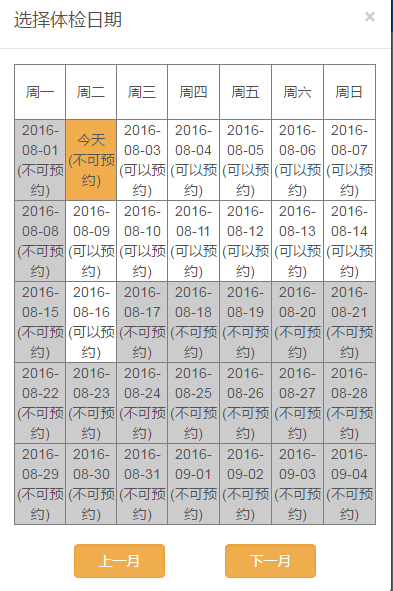# 移动端日期处理的一些问题

7533次浏览

## 相关知识

``````function getLocalTime(nS) {
var dataArray = new Date(parseInt(nS) * 1000).toLocaleDateString().split("/");
dataArray < 10 ? dataArray = "0" + dataArray : dataArray = dataArray;
dataArray < 10 ? dataArray = "0" + dataArray : dataArray = dataArray;
return dataArray.join("");
}``````

``Date.parse(str)``

``````function transformTime(str) {
str = str.replace(/-/g, '/');
var time = (new Date(str)).getTime();
return time / 1000;
}``````

## 案例说明``````function getLocalTime(nS) {
var dateStr=new Date(parseInt(nS) * 1000).toLocaleDateString();
dateStr=dateStr.replace(/年|月/g, "/").replace(/日/g,"");
var dataArray = dateStr.split("/");
dataArray < 10 ? dataArray = "0" + dataArray : dataArray = dataArray;
dataArray < 10 ? dataArray = "0" + dataArray : dataArray = dataArray;
return dataArray.join("");
}``````

``````function getLocalTime(nS) {
var dateStr=new Date(parseInt(nS) * 1000).toLocaleDateString();
dateStr=dateStr.replace(/年|月/g, "/").replace(/日/g,"");
var month = (new Date(dateStr)).getMonth() + 1;
var year = (new Date(dateStr)).getFullYear();
var date = (new Date(dateStr)).getDate();
if (month < 10) {
month = '0' + month;
}
if (date < 10) {
date = '0' + date;
}
return year + month + date;
}``````

PREVIOUS:
NEXT:

Tags: 移动端日期手机